# 6.01 Braids: Introduction

Below the video you will some pre-class questions and notes to accompany the video.

# Notes

## Definitions

(0.00)

Fix a collection of $$n$$ points $$z_1,\ldots,z_n$$ in $$\mathbf{C}$$. An $$n$$-strand braid $$F$$ is a collection of $$n$$ continuous maps $$F_1,\ldots,F_n\colon[0,1]\to\mathbf{C}$$ such that:
• $$F_i(t)\neq F_j(t)$$ if $$i\neq j$$
• $$F_i(0)=z_i$$, $$F_i(1)=z_{s(i)}$$ for some permutation $$s\colon\{1,\ldots,n\}\to\{1,\ldots,n\}$$.

We can draw a picture of a braid as a collection of pairwise disjoint paths $$\gamma_1,\ldots,\gamma_n$$ in $$\mathbf{C}\times[0,1]$$: $\gamma_k(t)=(F_k(t),t).$ In fact, since $$[0,1]$$ is compact and the image of a compact set by a continuous map is compact, the images of the paths $$F_k$$ are contained in some compact set in the plane, and we can always homotope everything (by a family of rescalings depending on $$t$$) to assume that our braids are contained in $$D\times[0,1]$$, where $$D$$ is the unit disc.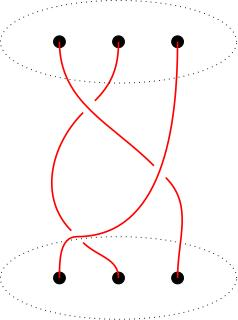The picture above shows a 3-strand braid in red which permutes the three black points via $$(13)$$ (if they are numbered $$1,2,3$$ left-to-right).

## Equivalence of braids

(3.23) We say that two $$n$$-strand braids $$F$$ and $$G$$ are equivalent if there is a collection of homotopies $$H_k(s,t)$$, $$k=1,\ldots,n$$, such that $$\{H_k(s,t)\}_{k=1}^n$$ is a braid for each fixed value of $$s$$ and such that $H_k(0,t)=F_k(t),\ H_k(1,t)=G_k(t),$

## Group law

(5.20) If $$F$$ and $$G$$ are two $$n$$-strand braids with associated permutations $$\sigma$$ and $$\tau$$ respectively then their product $$G\cdot F$$ is the braid $(G\cdot F)_i(t) =\begin{cases} F_i(2t)&\mbox{ if }t\in[0,1/2]\\ G_{s(i)}(2t-1)&\mbox{ if }t\in[1/2,1]. \end{cases}$

Pictorially, we multiply braids by stacking them: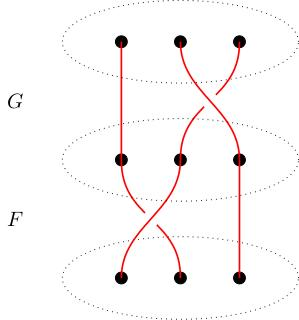The set of equivalence classes of $$n$$-strand braids form a group $$B_n$$ under this stacking product.
This is an exercise.

Much of the proof of this theorem should look a little bit like the proof that the fundamental group is a group. This is not a coincidence: the $$n$$-strand braid group is the fundamental group of a particular space, the unordered configuration space of $$n$$ points in the disc.

## Configuration space

(8.20) Let $$OC_n$$ be the subset of $$\mathbf{C}^n$$ defined by $OC_n:=\{(x_1,\ldots,x_n)\in \mathbf{C}^n\ :\ x_i\neq x_j\mbox{ for }i\neq h\}.$ We call a point $$(x_1,\ldots,x_n)\in OC_n$$ an ordered configuration of points in the disc and $$OC_n$$ is called the ordered configuration space of $$n$$ points in the plane. There is an action of the permutation group $$S_n$$ on $$OC_n$$; a permutation $$s$$ acts as $(x_1,\ldots,x_n)s=(x_{s(1)},\ldots,x_{s(n)}).$ The quotient $$UC_n:=OC_n/S_n$$ is called the space is called the unordered configuration space of $$n$$ points in the plane.

The fundamental group $$\pi_1(UC_n,[z_1,\ldots,z_n])$$ is isomorphic to the $$n$$-strand braid group.
This should be clear from the definition of a braid: a braid is a collection of paths $$F_1(t),\ldots, F_n(t)$$ with $$F_i(t)\neq F_j(t)$$ if $$i\neq j$$ and such that $$F_i(0)=z_i$$, $$F_i(1)=z_{s(i)}$$. Such a collection of paths defines a loop: $[F_1(t),\ldots,F_n(t)]$ in the unordered configuration space based at $$[z_1,\ldots,z_n]$$ and conversely. A homotopy of braids gives a homotopy of loops in the unordered configuration space (again, just by definition). Stacking braids corresponds to concatenating loops.

## Presentation of the braid group

(11.38) We will assume that the points $$z_i$$ are equally spaced along a line. For each $$i=1,2,\ldots,n-1$$ there is an elementary braid: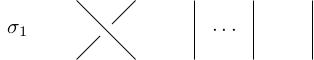,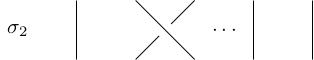$$\vdots$$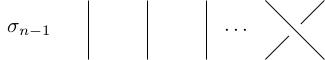The braid group $$B_n$$ is generated by the elementary braids subject to the following relations: \begin{gather*} \sigma_i\sigma_j=\sigma_j\sigma_i\mbox{ if }|i-j|\geq 1\\ \sigma_i\sigma_{i+1}\sigma_i=\sigma_{i+1}\sigma_i\sigma_{i+1}. \end{gather*}
Proof not included! It is an exercise to check that the braid relations hold. Later, I will give $$\epsilon$$ more explanation for how one would go about checking that these relations suffice.

# Pre-class questions

1. Why do braids form a group under stacking?